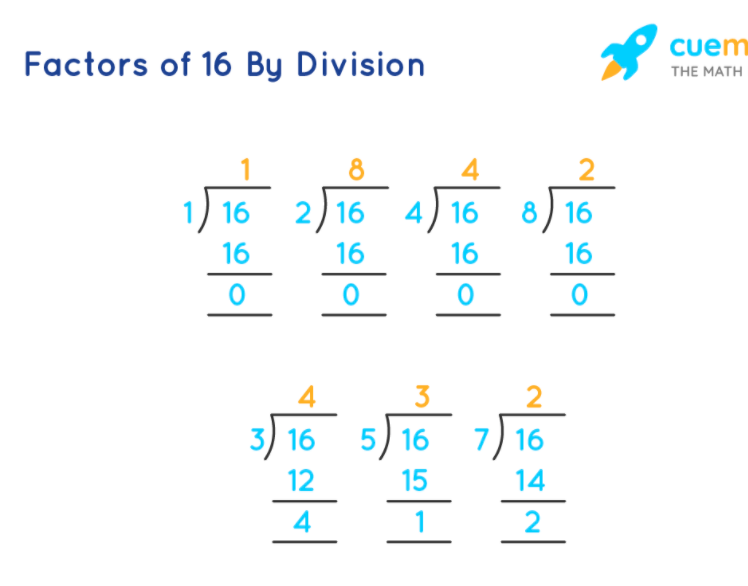# What Are All the Factors of 16

## Question: What are all the factors of 16?

A number that divides another number without leaving any remainder is called the factor of that number.

## Answer: The factors of 16 are 1, 2, 4, 8, and 16.

Let us find all the factors of 16.

## Explanation:

Using the division method, we can find the numbers which divide 16 exactly without leaving a remainder.